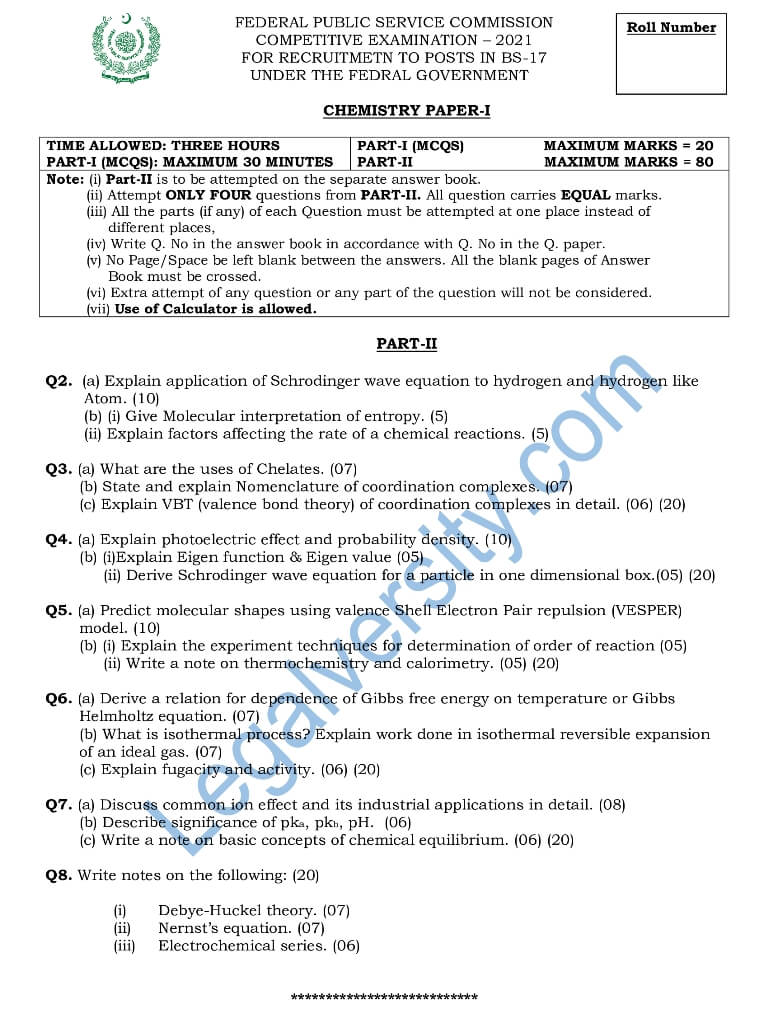# CSS Chemistry Paper-I 2021

We have here the CSS Chemistry Paper-I Paper 2021. You can view or download this CSS Chemistry Paper-I Paper 2021. The Chemistry paper-I of CSS was held on 25-02-2021.

### Get CSS Chemistry Paper-I Paper 2021

The following questions were given in the CSS Chemistry Paper-I Paper of 2021:

Q2.  (a) Explain application of Schrodinger wave equation to hydrogen and hydrogen-like
Atom. (10)
(b) (i) Give Molecular interpretation of entropy. (5)
(ii) Explain factors affecting the rate of chemical reactions. (5)

Q3. (a) What are the uses of Chelates. (07)
(b) State and explain the Nomenclature of coordination complexes. (07)
(c) Explain VBT (valence bond theory) of coordination complexes in detail. (06) (20)

Q4. (a) Explain photoelectric effect and probability density. (10)
(b) (i)Explain Eigenfunction & Eigenvalue (05)
(ii) Derive Schrodinger wave equation for a particle in a one-dimensional box. (05) (20)

Q5. (a) Predict molecular shapes using valence Shell Electron Pair repulsion (VESPER)
model. (10)
(b) (i) Explain the experimental techniques for the determination of the order of reaction (05)
(ii) Write a note on thermochemistry and calorimetry. (05) (20)

Q6. (a) Derive a relation for the dependence of Gibbs free energy on temperature or Gibbs
Helmholtz equation. (07)
(b) What is the isothermal process? Explain work done in the isothermal reversible expansion of an ideal gas. (07)
(c) Explain fugacity and activity. (06) (20)

Q7. (a) Discuss common ion effects and their industrial applications in detail. (08)
(b) Describe the significance of pka, pkb, pH.  (06)
(c) Write a note on basic concepts of chemical equilibrium. (06) (20)

Q8. Write notes on the following: (20)

• Debye-Huckel theory. (07)
• Nernst’s equation. (07)
• Electrochemical series. (06)Other CSS (Compulsory) 2021 Papers:

Other CSS (Optional) 2021 Papers:

➤➤ View All CSS Papers of 2021 here

You may also like these:

Subscribe
Notify of